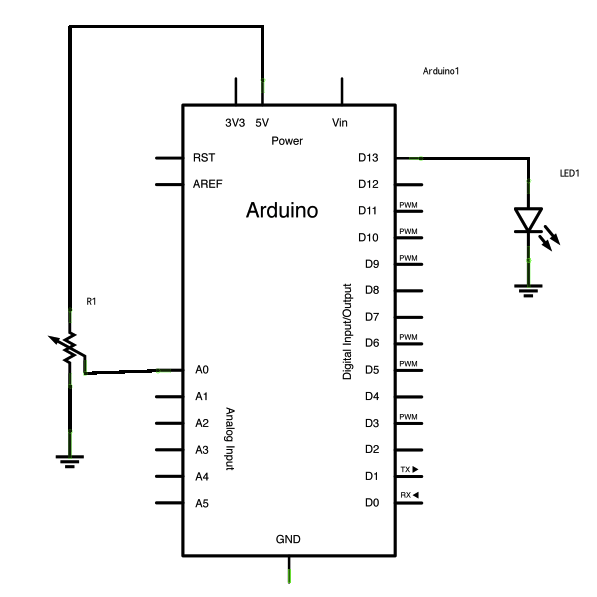# Potentiometer or variable resistor control LED Code

The if() statement is the most basic of all programming control structures. It allows you to make something happen or not depending on whether a given condition is true or not. It looks like this:if (someCondition) {
// do stuff if the condition is true
}

There is a common variation called if-else that looks like this:

if (someCondition) {
// do stuff if the condition is true
} else {
// do stuff if the condition is false
}

There’s also the else-if, where you can check a second condition if the first is false:

if (someCondition) {
// do stuff if the condition is true
} else if (anotherCondition) {
// do stuff only if the first condition is false
// and the second condition is true
}

You’ll use if statements all the time. The example below turns on an LED on pin 13 (the built-in LED on many Arduino boards) if the value read on an analog input goes above a certain threshold.

### Circuit

image developed using Fritzing. For more circuit examples, see the Fritzing project page

Schematic:### Code

In the code below, a variable called `analogValue` is used to store the data collected from a potentiometer connected to the Arduino on `analogPin 0`. This data is then compared to a threshold value. If the analog value is found to be above the set threshold the LED connected to digital pin 13 is turned on. If analogValue is found to be `<` threshold, the LED remains off.

### Hardware Required

• Arduino Board
• (1) Potentiometer or variable resistor
• (1) 220 ohm resistor
• (1) LED
• hook-up wire

For more detail: Potentiometer or variable resistor control LED Code

#### This Post / Project can also be found using search terms:

• arduino led projects
• led potentiometer dimmer code
• how to set threshold on Arduino based off resistance
• Arduino rgb control with pot